# 2D Phase Shifter for Modulator Design

## Tools Used: TCAD, BeamPROP

This application note demonstrates the simulation of a 2D phase shifter cross-section to be used in a modulator. The phase shifter simulation is entirely run via Synopsys’ Sentaurus Workbench (SWB), allowing the project to be easily shared between various functional groups in an organization. For example, one group could author the S-Process step, and another the S-Device and optical steps. Each group can then experiment with the entire project without needing a full understanding of all steps.

The output of the modal effective index vs. applied voltage, effective index vs. wavelength, and frequency response of a 2D phase shifter for modulator design is demonstrated. These are typical parameters used to build a compact model for the phase shifter, other parameters can be calculated/extracted as needed.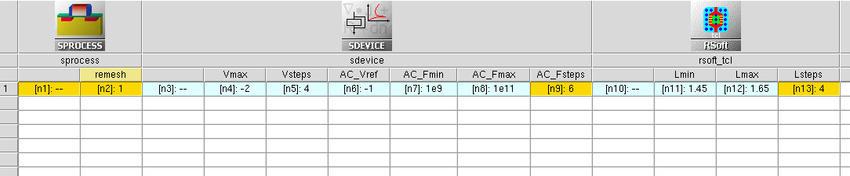Figure 1. The SWB workflow (left to right).

The three main steps to the simulation flow are:

Step 1: Create the structure using S-Process

Step 2: S-Device is used to calculate the electron and hole distributions at various applied voltages and frequencies.

Step 3: The RSoft Photonic Device Tools mode solver, BeamPROP, is used to calculate the modal complex effective index, and output the effective index vs. applied voltage, effective index vs. wavelength, and frequency response plots.

## Step 1: Creating the Structure in S-Process

The phase shifter consists of a standard Si rib waveguide in an oxide background. Boron implants are placed in the device, resulting in the doping profile shown below. Two electrical contacts (not shown) are included on the left and right of the waveguide.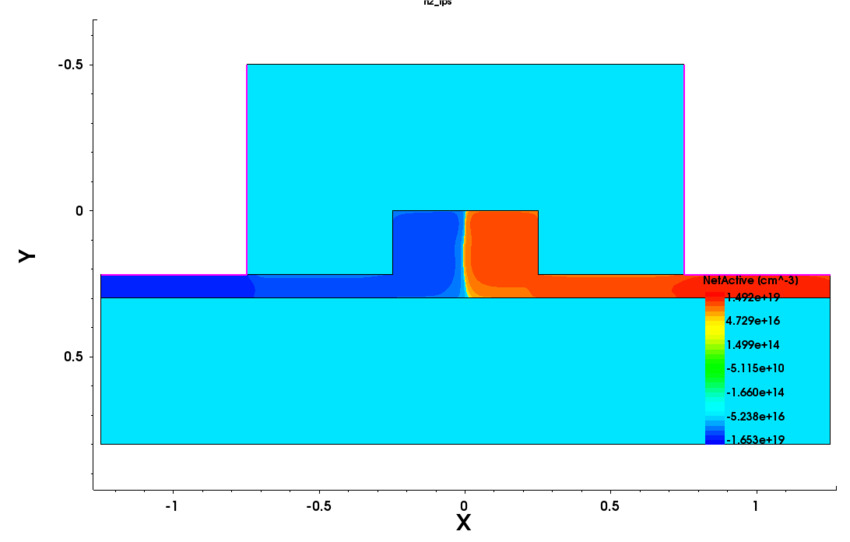Figure 2. The Sentaurus TCAD S-Process structure and doping of the 2D cross-section of the phase shifter.

## Step 2: Calculate Electron/Hole Densities with S-Device

The S-Device step takes the structure and doping profile from S-Process, and calculates the electron/hole densities at various voltages and frequencies. As configured, 5 voltage points are used (-2.0 to 0V in ½ volt increments) and 7 frequencies (10e9 and 10e11 with7 points, logarithmically spaced). These 12 outputs are used in the Step 3 to determine the optical performance of the phase shifter.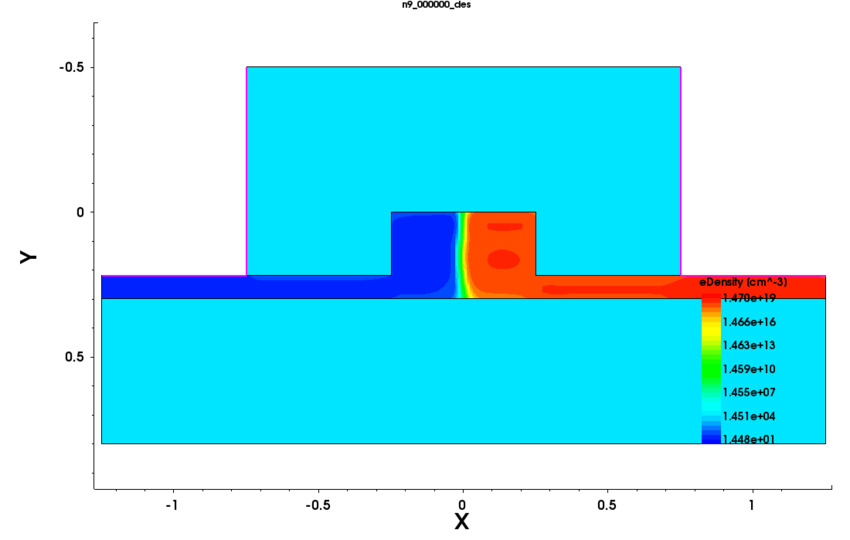Figure 3. Example electron distribution for one specific voltage.

## Step 3: Calculate the Optical Performance

The BeamPROP mode solver is used to calculate the mode of the structure (including its effective index) at each Voltage/Frequency from Step 2. The following 3 outputs are then generated.

• Effective Index vs. Voltage
• Effective Index vs. Wavelength
• Frequency Response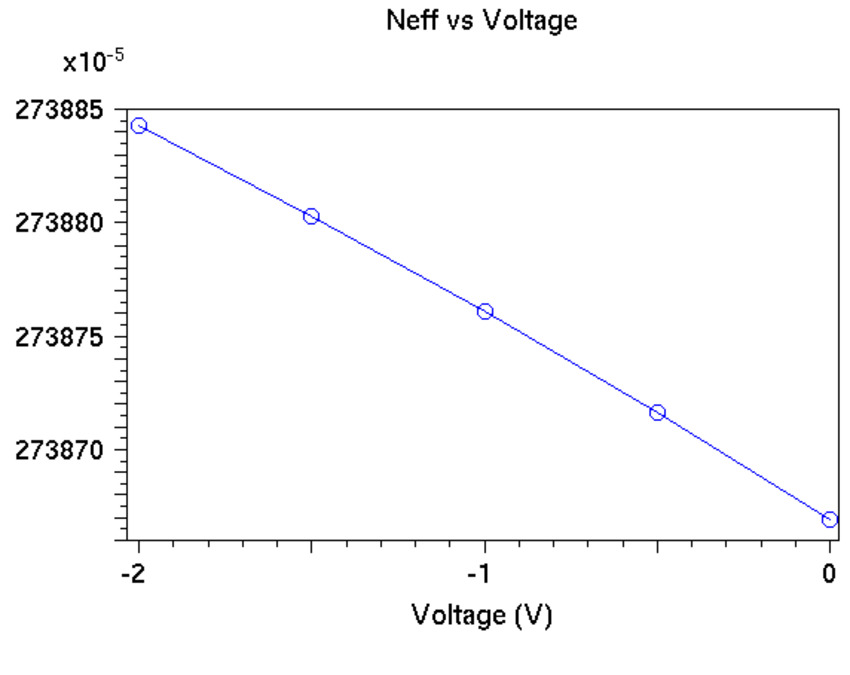Figure 4. Real Effective Index vs. Voltage. The imaginary part of the effective index can also be displayed, and is used to calculate loss.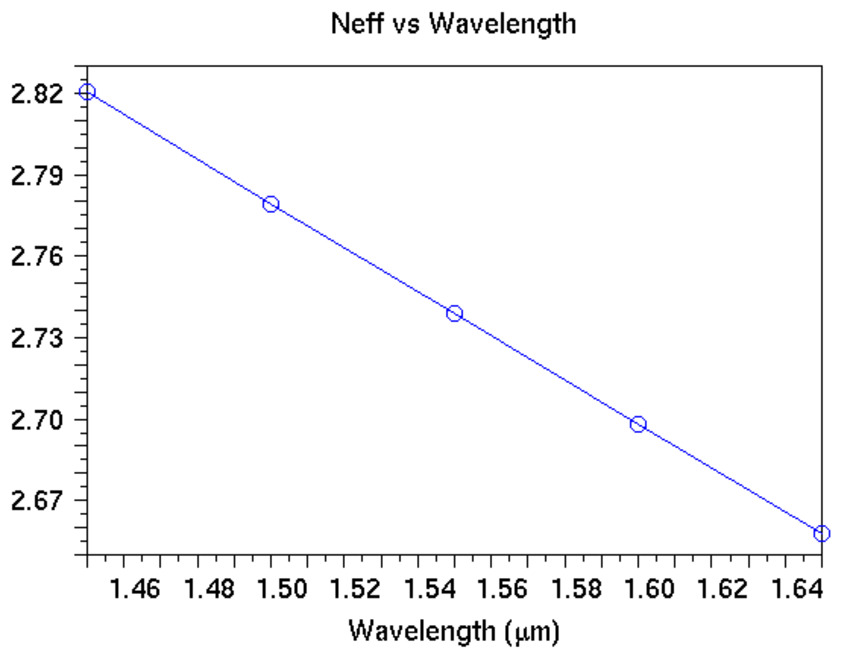Figure 5. Real Effective Index vs. Wavelength.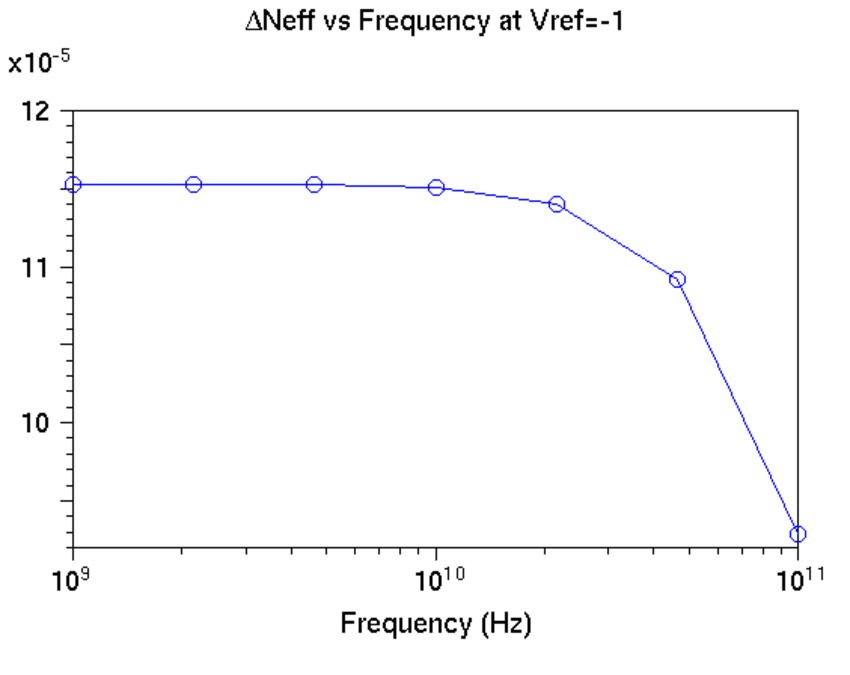Figure 6. ΔNeff vs. Frequency